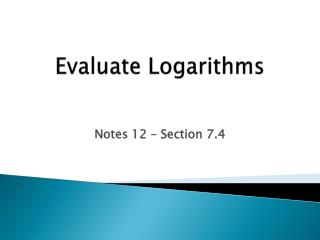DownloadDownload PresentationEvaluate Logarithms

# Evaluate Logarithms

Download Presentation## Evaluate Logarithms

- - - - - - - - - - - - - - - - - - - - - - - - - - - E N D - - - - - - - - - - - - - - - - - - - - - - - - - - -
##### Presentation Transcript

1. Evaluate Logarithms Notes 12 – Section 7.4

2. Essential Learnings • Students will understand and be able to evaluate exponential functions using logarithms. • Students will be able to recognize and use equivalent representations of expressions.

3. Logarithm with Base b Let band ybe positive numbers with b≠1. The logarithm of ywith base b is defined as follows: if and only if “Log base b of y”

4. Common Logarithm Logarithm with base 10: Logarithm with base e: “natural log”

5. Example 1 (3-6) Rewrite the logarithm into exponential form.

6. Example 2 (3-6) Rewrite the logarithm into exponential form.

7. Example 3 (3-6) Rewrite the logarithm into exponential form.

8. Class Problems Rewrite each logarithm into exponential form.

9. Example 4 (8-19) Evaluate the logarithm without a calculator (change from logarithmic form to exponential form).

10. Example 5 (8-19) Evaluate the logarithm without a calculator (change from logarithmic form to exponential form).

11. Example 6 (8-19) Evaluate the logarithm without a calculator (change from logarithmic form to exponential form).

12. Class Problems Evaluate the logarithm without a calculator (change from logarithmic form to exponential form).

13. Calculating Logarithms (20-27) Use a calculator to evaluate the logarithm.

14. Exponential vs. Logarithmic • How are logarithmic functions related to exponential functions? They are inverse functions.

15. Inverse Properties of Logarithms

16. Example 7 (29-35) Simplify the expressions using inverse properties.

17. Example 8 (29-35) Simplify the expressions using inverse properties. The log is base 2, so change 16 into 24 to simplify.

18. Example 9 (29-35) Simplify the expressions using inverse properties. The log is base 3, so change 81 into 34 to simplify.

19. Assignment Pages 503: 3–6, 8–19, 20, 23, 24, 26, 27, 29–35 odd MM 3-1 – Due Tuesday/Wednesday Tuesday ICE – Finish Mastery Assignment Unit Study Guide 5 – Keep working on it!